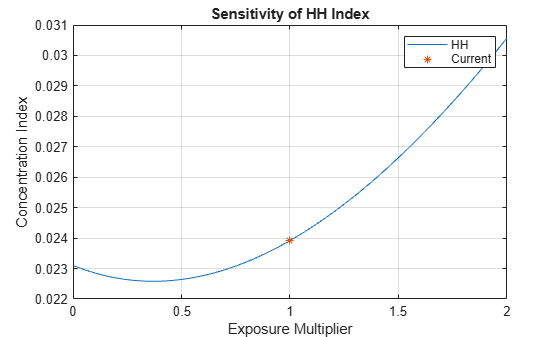Documentation

## Analyze the Sensitivity of Concentration to a Given Exposure

This example shows how to sweep through a range of values for an existing exposure from `0` to double the current value and plot the corresponding values. This could be used as one criterion (among others) for assessing portfolio limits.

Load credit portfolio data and use exposure at default (`EAD`) as the portfolio values. Compute current values of concentration indices.

```load CreditPortfolioData.mat P = EAD; CurrentConcentration = concentrationIndices(P)```
```CurrentConcentration=1×8 table ID CR Deciles Gini HH HK HT TE ___________ ________ _____________ _______ ________ ________ ________ _______ "Portfolio" 0.058745 [1x11 double] 0.55751 0.023919 0.013363 0.022599 0.53485 ```

Choose an index of interest. For instance, select a loan with maximum exposure.

```[~,IndMax] = max(P); CurrentExposure = P(IndMax);```

Sweep through a range of multipliers for the selected exposure and get the corresponding concentration measures.

```Multiplier = 0.0:0.05:2; % Compute concentration with selected exposure removed from portfolio P(IndMax) = 0; ciSensitivity = concentrationIndices(P,'ID','Multiplier 0.0'); ciSensitivity = repmat(ciSensitivity,length(Multiplier),1); for ii=2:length(Multiplier) P(IndMax) = CurrentExposure*Multiplier(ii); ci = concentrationIndices(P,'ID',['Multiplier ' num2str(Multiplier(ii))]); ciSensitivity(ii,:) = ci; end % Display first five rows disp(ciSensitivity(1:5,:))```
``` ID CR Deciles Gini HH HK HT TE _________________ ________ _____________ _______ ________ ________ ________ _______ "Multiplier 0.0" 0.059442 [1x11 double] 0.55051 0.023102 0.013314 0.022248 0.51826 "Multiplier 0.05" 0.059257 [1x11 double] 0.5467 0.022968 0.013185 0.022061 0.50991 "Multiplier 0.1" 0.059074 [1x11 double] 0.54456 0.022855 0.013156 0.021957 0.5059 "Multiplier 0.15" 0.058891 [1x11 double] 0.54355 0.022762 0.013143 0.021908 0.50352 "Multiplier 0.2" 0.058709 [1x11 double] 0.54313 0.022688 0.013139 0.021888 0.5022 ```

Plot the sensitivity to changes in exposure for a particular index.

```IndexID = 'HH'; figure; plot(Multiplier',ciSensitivity.(IndexID)) hold on plot(1,CurrentConcentration.(IndexID),'*') hold off title(['Sensitivity of ' IndexID ' Index']) xlabel('Exposure Multiplier') ylabel('Concentration Index') legend(IndexID,'Current') grid on```